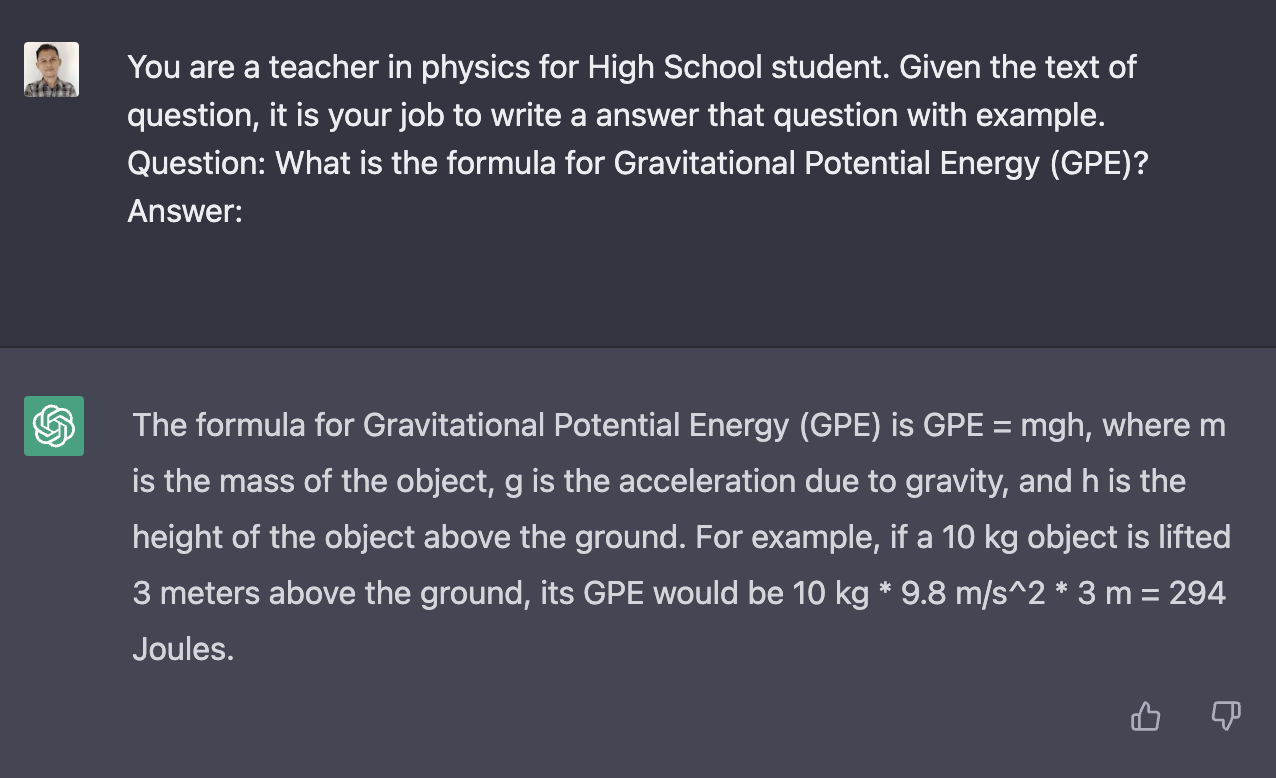# How to replicate ChatGPT with Langchain and GPT-3?self.__wrap_balancer=(t,e,n)=>{n=n||document.querySelector(`[data-br="\${t}"]`);let o=n.parentElement,r=E=>n.style.maxWidth=E+"px";n.style.maxWidth="";let i=o.clientWidth,s=o.clientHeight,c=i/2,u=i,d;if(i){for(;c+1<u;)d=~~((c+u)/2),r(d),o.clientHeight==s?u=d:c=d;r(u*e+i*(1-e))}};self.__wrap_balancer(":R2sm:",1)

It is well-known that ChatGPT is currently capable of impressive feats. It is likely that many individuals have ideas for utilizing this technology in their own projects. However, it should be noted that ChatGPT does not currently have an official API. Using an unofficial API may result in difficulties.

At present, it is necessary to manually obtain an access token and cloudflare token in order to use the API. Furthermore, these tokens must be manually changed every two hours.

## ChatGPT

ChatGPT utilizes GPT-3 models in its design, and a new model has been developed based on this. Therefore, the output of the new model is often similar to that of GPT-3. At the time of writing, the `text-davinci-002-render` model was used in the ChatGPT of the new model, but it is not currently accessible to the public.

While ChatGPT may not be groundbreaking, it offers a new interface for utilizing existing technologies. By utilizing a strong prompt and an efficient memory window. So, instead of hacking the unofficial API of ChatGPT, we can replicate its capabilities using the LLM Chain method.

## LangChain

Langchain is new python package it provides a standard interface for chains, lots of integrations with other tools, and end-to-end chains for common applications.

LangChain is designed to assist in four main areas, which are listed here in increasing order of complexity:

1. LLM and Prompts
2. Chains
3. Agents
4. Memory

## Install

To use langchain package you can install it from pypi.

``````pip install langchain
``````

To get latest update from langchain, you can use this installation method.

``````pip install "git+https://github.com/hwchase17/langchain.git"
``````

## Example Project

There's a lot you can do with ChatGPT one of the interesting one is to build Q&A for student homework. So this time we will create AI version of Brainly.

This is what we'll get from ChatGPT.Here's the prompt with langchain.

``````from langchain.llms import OpenAI
from langchain.chains import LLMChain
from langchain.prompts import PromptTemplate
from langchain.chains import SimpleSequentialChain

llm = OpenAI(temperature=.7)
template = """You are a teacher in physics for High School student. Given the text of question, it is your job to write a answer that question with example.
Question: {text}
"""
prompt_template = PromptTemplate(input_variables=["text"], template=template)
``````

And here's the result we will get from GPT-3 with langchain.

``The formula for Gravitational Potential Energy (GPE) is GPE = mgh, where m is the mass of an object, g is the acceleration due to gravity, and h is the height of the object. For example, if an object with a mass of 10 kg is at a height of 5 meters, then the GPE would be GPE = 10 x 9.8 x 5 = 490 Joules.``

## Chatbot

If you need to create chatbot like AI you can use memory with langchain. Here's example how to do it.

``````from langchain.chains.conversation.memory import ConversationBufferMemory
from langchain import OpenAI, LLMChain, PromptTemplate

template = """You are a teacher in physics for High School student. Given the text of question, it is your job to write a answer that question with example.
{chat_history}
Human: {question}
AI:
"""
prompt_template = PromptTemplate(input_variables=["chat_history","question"], template=template)
memory = ConversationBufferMemory(memory_key="chat_history")

llm_chain = LLMChain(
llm=OpenAI(),
prompt=prompt_template,
verbose=True,
memory=memory,
)

llm_chain.predict(question="What is the formula for Gravitational Potential Energy (GPE)?")

result = llm_chain.predict(question="What is Joules?")
print(result)
``````

And the result would be like this.

``````\$ python3 memory.py

> Entering new LLMChain chain...
Prompt after formatting:
You are a teacher in physics for High School student. Given the text of question, it is your job to write a answer that question with example.

Human: What is the formula for Gravitational Potential Energy (GPE)?
AI:

> Finished LLMChain chain.

> Entering new LLMChain chain...
Prompt after formatting:
You are a teacher in physics for High School student. Given the text of question, it is your job to write a answer that question with example.

Human: What is the formula for Gravitational Potential Energy (GPE)?
AI:
The formula for Gravitational Potential Energy (GPE) is GPE = mgh, where m is the mass of the object, g is the acceleration due to gravity, and h is the height of the object.

For example, if an object has a mass of 10 kg and is at a height of 5 meters, then the gravitational potential energy of the object is GPE = 10 kg x 9.8 m/s2 x 5 m = 490 Joules.
Human: What is Joules?
AI:

> Finished LLMChain chain.
Joules (J) is the SI unit of energy. It is defined as the amount of energy required to move an object of one kilogram at a speed of one meter per second. It is also equal to the work done when a force of one Newton is applied to an object and moved one meter in the direction of the force.
``````

## Conclusion

ChatGPT is a GPT-3 based chatbot and currently does not have an official API. Using LangChain, developers can replicate the capabilities of ChatGPT, such as creating chatbots or Q&A systems, without having to use the unofficial API.

LangChain provides a standard interface, lots of integrations, and end-to-end chains for common applications. It can be installed from pypi and more information can be found in the official documentation.

If you have any question let me know, you can contact me on twitter.

Built with Next.js, Tailwind and Vercel